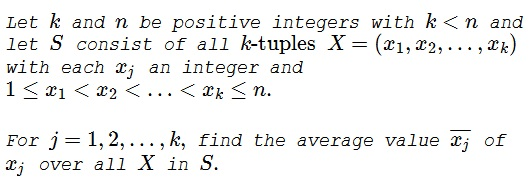# Averages of Terms in Increasing Sequence

### Problem### Solution 1

The number of $k\text{-tuples}$ $X$ in which $x_j=m$ is $\displaystyle {m-1\choose j-1}{n-m\choose k-j}.$ Indeed, we are free to choose $j-1$ numbers from the first $m-1$ and $k-j$ numbers from among $m+1,\ldots,n.$ Thus, the total number of $k\text{-tuples}$ equals

$\displaystyle \sum_{m=j}^{n-k+j}{m-1\choose j-1}{n-m\choose k-j}={n\choose k}.$

For the average then

\displaystyle\begin{align} \overline{x_j}&= \sum_{m=j}^{n-k+j}m{m-1\choose j-1}{n-m\choose k-j}\bigg/{n\choose k}\\ &=\sum_{m=j}^{n-k+j}j{m\choose j}{n-m\choose k-j}\bigg/{n\choose k}\\ &=j\sum_{m=j}^{n-k+j}{(m+1)-1\choose (j+1)-1}{(n+1)-(m+1)\choose (k+1)-(j+1)}\bigg/{n\choose k}\\ &=j\sum_{m'=j\,'}^{n-k+j\,'}{m'-1\choose j\,'-1}{(n+1)-m'\choose (k+1)-j\,'}\bigg/{n\choose k}\\ &=j\cdot {n+1\choose k+1}\bigg/{n\choose k}\\ &=j\cdot\frac{n+1}{k+1}, \end{align}

where $m'=m+1$ and $j\,'=j+1.$

### Solution 2

First we use the bijection

\begin{alignat*}{3} \phi :& S &\to& S \\ &(x_1,...,x_k) &\mapsto& (x_2-x_1,...,x_n-x_1,n+1-x_1) \end{alignat*}

\begin{align*} \bar{x}_1 =& \frac{1}{S}\sum _{x\in S} x_1\\ =& \frac{1}{S}\sum _{x\in S} \phi(x) _1\\ =& \frac{1}{S}\sum _{x\in S} x_2 - x_1 \\ =& \bar{x} _{2} - \bar{x} _{1} \end{align*}

Likewise we obtain $\bar{x} _{j+1} - \bar{x} _j = \bar{x} _1$ by using $\phi^j$ instead of $\phi$ where $j = 1,...,k-1.$ This shows that

(1)

$\begin{equation} \bar{x} _{j} = j\bar{x}_1 \quad\text{ for } j = 1,...,k \label{eq:1} \end{equation}$

Now consider the bijection

\begin{alignat*}{3} \psi :& S &\to& S \\ &(x_1,...,x_k) &\mapsto& (n+1-x_k,...,n+1-x_1) \end{alignat*}

Summation is commutative, so we get for each $j =1,...,k :$

\begin{align*} \bar{x}_1 =& \frac{1}{S}\sum _{x\in S} x_1\\ =& \frac{1}{S}\sum _{x\in S} \psi(x) _1\\ =& \frac{1}{S}\sum _{x\in S} n+1-x _{k}\\ =& n+1-\bar{x} _{k}\\ =& n+1-k\bar{x} _1 \quad\text{by (1)} \end{align*}

From which we conclude $\displaystyle \bar{x}_1 = \frac{n+1}{k+1} .$

### Solution 3

For the number of different such sequences, consider $k$ red 1s, $n-k$ blue $1s$, each of which may be placed at one if the $k+1$ locations between the red 1s. This gives ${n\choose k}$ such sequences. But this shows us more.

There is only one location for blue 1s that increases the value of $x_1,$ but two for $x_2,$ etc. Thus $E(x_i) = i\cdot E(x_1).$ What is $E(x_1)?$

Think of the value of each $x_i$ as equal to the number of red+blue $1s$ up to and including the $i^{th}$ red $1.$ Each blue $1$ has $k+1$ locations (before the first red $1,$ between the first and second red $1$ etc.) The first location increments all $x_i;$ the last none.

Thus the expected value each adds to $x_1+\ldots+x_k$ is the average of $0, 1, \ldots, k,$ or $\displaystyle \frac{k}{2}.$

Thus, each blue 1 adds an expected value of $\displaystyle \frac{k}{2}$ to $\sum E(x_i),$ so $\displaystyle E(x_1)\cdot k\cdot \frac{k+1}{2} = k\cdot\frac{k+1}{2}+(n-k)\cdot \frac{k}{2},$ thus $\displaystyle E(x_1) = 1+\frac{n-k}{k+1} = \frac{n+1}{k+1}$ and so $\displaystyle E(x_j) = j\cdot \frac{n+1}{k+1}.$

### Solution 4

Casting it as $k+1$ sequential urns makes it visually clearer. The value of $x_i$ is $i + \text{the sum of the balls in urns } 1, \ldots, i$ and we're placing $n-k$ balls in the $k+1$ urns.

The probability each ball increases the value of $x_i$ is $\displaystyle \frac{i}{k+1}$ (placed in urn $x_1, \dots, x_i),$ hence the expected value of $E(x_i)$ is immediately $\displaystyle i+(n-k)\cdot \frac{i}{k+1} = i\cdot \frac{n+1}{k+1}.$

### Acknowledgment

This is problem B-425 from The Fibonacci Quarterly (1980,18(2)). The problem is by Richard M. Grassl, Solution 1 is by Graham Lord.

Solution 2 is by Long Huynh Huu. Solutions 3 and 4 are by Christopher D. Long.